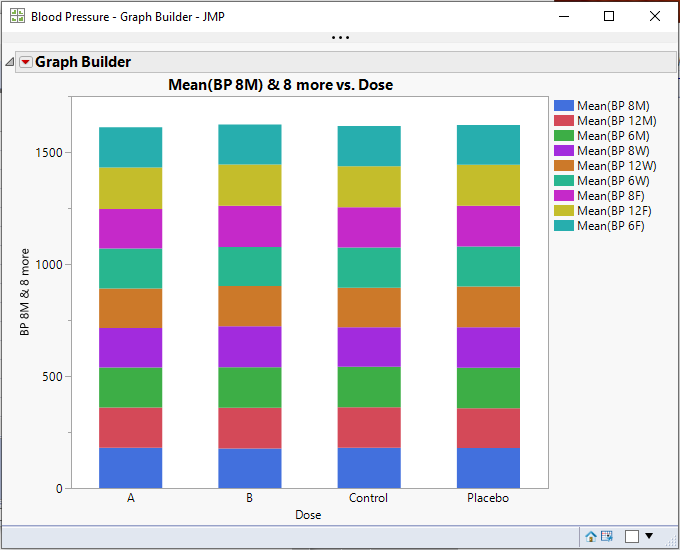Choose Language Hide Translation Bar
Highlighted

## Graph Builder

Hi, im new to the jmp scripting language. The code below works(it shows bar graphs of the column 3 to n) but there is one thing i'd want to do. How do i script such that my output should be a stacked bar chart of all columns?

for(i=3,i<=NItems(colList2),i++,

gb= dt4 << Graph Builder(

Size(534,454),

Show Control Panel(0),

Variables(

X(:Label),Y(eval(Column(dt4,colList2[i]))),

),

Elements(Bar(X,Y))

));

1 ACCEPTED SOLUTION

Accepted Solutions
Highlighted

## Re: Graph Builder

There is not a single statement that can be expanded on to cover a different number of incoming Y columns.  Therefore, you need to take the basic form, and dynamically create it depending upon the number of columns

Here is the basic form

``````Graph Builder(
Size( 534, 450 ),
Show Control Panel( 0 ),
Variables(
X( :SITE ),
Y( :NPN1 ),
Y( :PNP1, Position( 1 ) ),
Y( :PNP2, Position( 1 ) ),
Y( :NPN2, Position( 1 ) ),
Y( :PNP3, Position( 1 ) )
),
Elements(
Bar(
X,
Y( 1 ),
Y( 2 ),
Y( 3 ),
Y( 4 ),
Y( 5 ),
Legend( 4 ),
Bar Style( "Stacked" )
)
)
);``````

Here is the Example script that I created that dynamically builds a stacked bar chart

``````names default to here(1);
dt=open("\$SAMPLE_DATA/Blood Pressure.jmp");

// Get all of the continuous data columns
colList = dt << get column names(continuous,string);

// Create the JSL to create the script
theExpr = "Graph Builder(
Size( 534, 450 ),
Show Control Panel( 0 ),
Variables(
X( :Dose ),";

// Add the first Y entry
theExpr = theExpr || "Y( :" || colList || " )";

// Add the remaining Y entries
For( i=2, i<= n items(colList), i++,
theExpr = theExpr || ", Y( :" || colList[i] || ", Position( 1 ) )";
);

// Finish up the Variables list, and start the Elements
theExpr = theExpr || "), Elements( Bar(X, Y( 1 )";

// Add the remaining Y elements
For( i=2, i<= n items(colList), i++,
theExpr = theExpr || ", Y( " || char(i) || " )";
);

// Finish up the required specifications
theExpr = theExpr || ",
Legend( 4 ),
Bar Style( \!"Stacked\!" )
)
)
);";

// Run the created JSL
eval(parse(theExpr));``````

That producesJim
3 REPLIES 3
Highlighted

## Re: Graph Builder

Interactively, go to Graph Builder, and develop the graph to look the way you want it to look, and then just simply go to the red triangle, and select "Save Script". JMP creates the JSL for you.
Jim
Highlighted

## Re: Graph Builder

Thank you for the reply! Do you have any idea how i should do it for the script to automatically run the script in different data sets with different column names without having to use the graph builder interactively?

@txnelson wrote:
Interactively, go to Graph Builder, and develop the graph to look the way you want it to look, and then just simply go to the red triangle, and select "Save Script". JMP creates the JSL for you.

Highlighted

## Re: Graph Builder

There is not a single statement that can be expanded on to cover a different number of incoming Y columns.  Therefore, you need to take the basic form, and dynamically create it depending upon the number of columns

Here is the basic form

``````Graph Builder(
Size( 534, 450 ),
Show Control Panel( 0 ),
Variables(
X( :SITE ),
Y( :NPN1 ),
Y( :PNP1, Position( 1 ) ),
Y( :PNP2, Position( 1 ) ),
Y( :NPN2, Position( 1 ) ),
Y( :PNP3, Position( 1 ) )
),
Elements(
Bar(
X,
Y( 1 ),
Y( 2 ),
Y( 3 ),
Y( 4 ),
Y( 5 ),
Legend( 4 ),
Bar Style( "Stacked" )
)
)
);``````

Here is the Example script that I created that dynamically builds a stacked bar chart

``````names default to here(1);
dt=open("\$SAMPLE_DATA/Blood Pressure.jmp");

// Get all of the continuous data columns
colList = dt << get column names(continuous,string);

// Create the JSL to create the script
theExpr = "Graph Builder(
Size( 534, 450 ),
Show Control Panel( 0 ),
Variables(
X( :Dose ),";

// Add the first Y entry
theExpr = theExpr || "Y( :" || colList || " )";

// Add the remaining Y entries
For( i=2, i<= n items(colList), i++,
theExpr = theExpr || ", Y( :" || colList[i] || ", Position( 1 ) )";
);

// Finish up the Variables list, and start the Elements
theExpr = theExpr || "), Elements( Bar(X, Y( 1 )";

// Add the remaining Y elements
For( i=2, i<= n items(colList), i++,
theExpr = theExpr || ", Y( " || char(i) || " )";
);

// Finish up the required specifications
theExpr = theExpr || ",
Legend( 4 ),
Bar Style( \!"Stacked\!" )
)
)
);";

// Run the created JSL
eval(parse(theExpr));``````

That producesJim
Article Labels

There are no labels assigned to this post.